# Why are neural networks so powerful?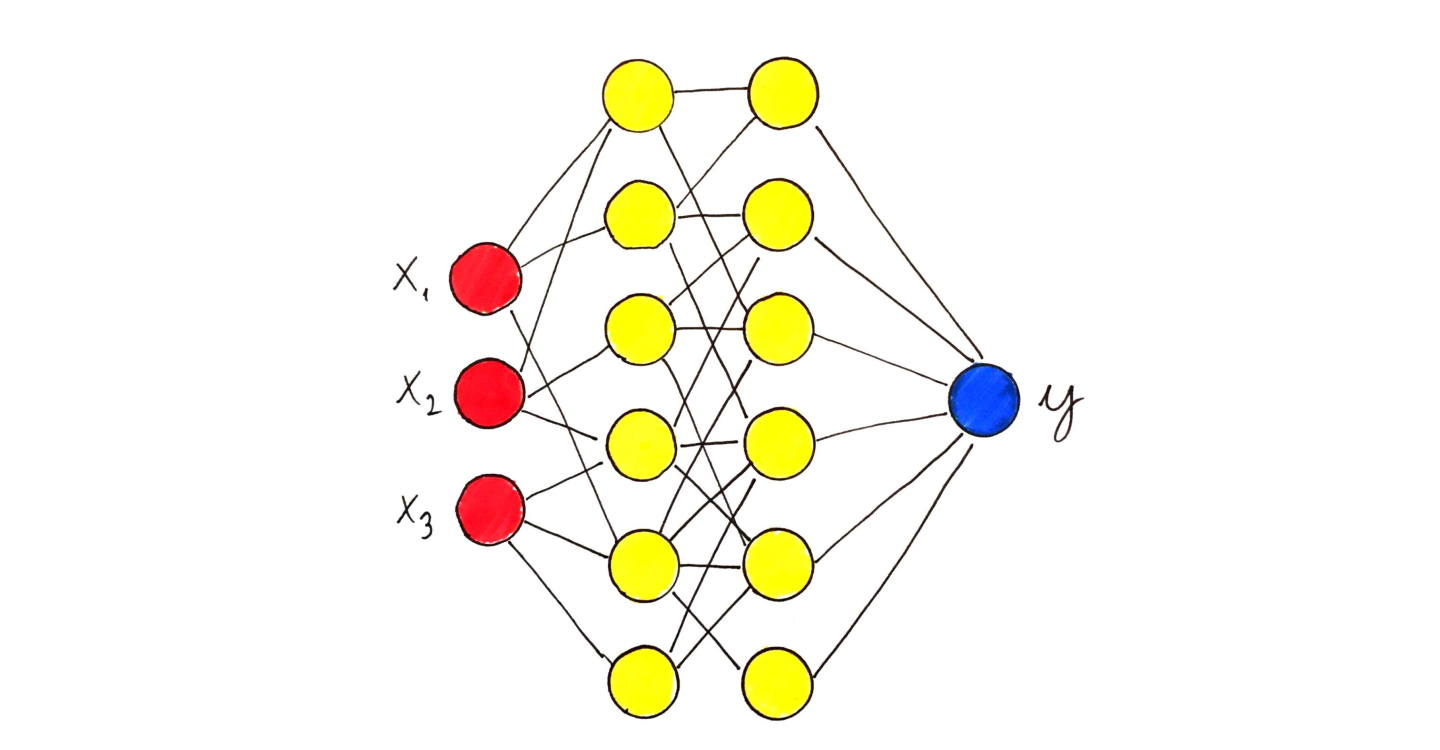It is common knowledge that neural networks are very powerful, and they can be used for almost any statistical learning problem with excellent results. But have you thought about why this is the case? Why is this method more potent in most scenarios than many other algorithms?

As always with machine learning, there is a precise mathematical reason for this. Simply saying, the set of functions described by a neural network model is extensive. But what does describing a set of functions mean? How can a set of functions be large? These concepts seem difficult to grasp at first glance. However, they can be properly defined, shedding light on why certain algorithms are better than others.

## Machine learning as function approximation

Let's take an abstract viewpoint and formulate what a machine learning problem is. Suppose we have our datasetwhere x⁽ᵏ⁾ is a data point and y⁽ᵏ⁾ is the observation related to the data point. The observation y⁽ᵏ⁾ can be a real number or even a probability distribution (in the case of classification). The task is simply to find a function f(x) for which f(x⁽ᵏ⁾) is approximately y⁽ᵏ⁾.

For this, we fix a parametrized family of functions in advance and select a parameter configuration that has the best fit. For instance, linear regression uses the function familyas a parametric family of functions, with a and b as parameters.

If we suppose a true underlying function g(x) that describes the relationship between x⁽ᵏ⁾ and y⁽ᵏ⁾, the problem can be phrased as a function approximation problem. This leads us into the beautiful albeit very technical field of approximation theory.

## A primer on approximation theory

Probably you have encountered the exponential function throughout your life several times. It is defined bywhere e is the famous Euler number. This is a transcendental function, which means that you cannot calculate its value with finitely many additions and multiplications. However, when you punch this into a calculator, you'll still get a value. This value is an approximation only, although it is often sufficient for our purposes. In fact, we havewhich is a polynomial, so its value can be calculated explicitly. The larger n is, the closer the approximation to the actual value.

The central problem of approximation theory is to provide a mathematical framework for these problems. If you have any function g(x) and a family of functions that are easier to handle from computational aspects, your goal is to find a "simple" function close enough to g. In essence, approximation theory searches for answers to three core questions.

• What is "close enough"?
• Which family of functions can (or should) I use to approximate?
• From a given approximating function family, which exact function is the one that will fit the best?

Don't worry if these sound a bit abstract because we will look into the special case of neural networks next.

## Neural networks as function approximators

So, let's reiterate the problem. We have a function g(x) that describes the relation between data and observation. This is not known exactly, only for some valueswhere g(x⁽ᵏ⁾) = y⁽ᵏ⁾. Our job is to find an f(x) which

• generalizes the knowledge from the data,
• and computationally feasible.

If we assume that all of our data points are in the subset X, that isholds, we would like a function where the quantity supremum norm defined byis as small as possible. You can imagine this quantity by plotting these functions, coloring the area enclosed by the graph, and calculating the maximum spread of said area along the y axis.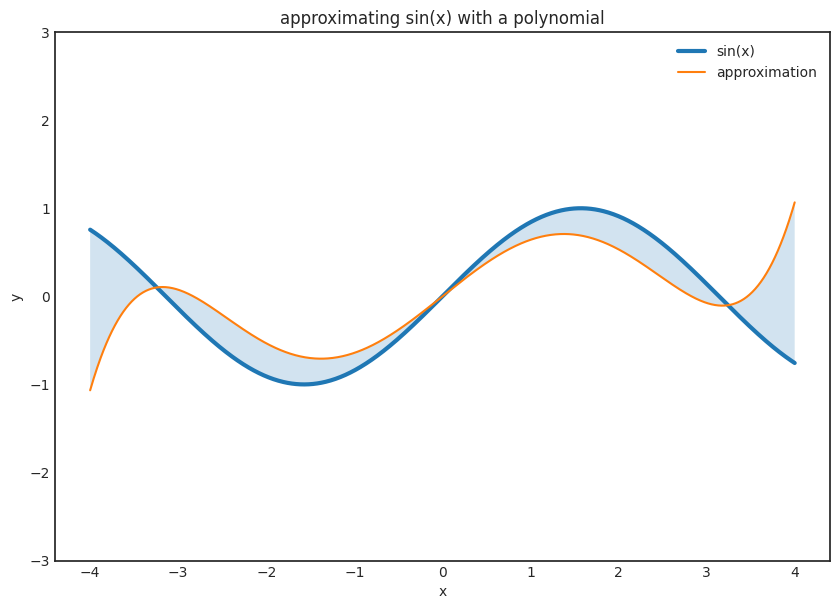Even though we cannot evaluate g(x) for arbitrary values, we should always aim to approximate it in this broader sense, instead of requiring f(x) to fit only at the known data points xₖ. So, the problem is given. The question is, which set of functions should we use to approximate with?

## Neural networks with a single hidden layer

Mathematically speaking, a neural network with a single hidden layer is defined bywhere φ is a nonlinear function (called activation function) such as the Sigmoid functionandThe value x corresponds to the data, while wᵢ, bᵢ and vᵢ are the parameters. Is the function familyenough to approximate any reasonable function? The answer is a resounding yes!

## The universal approximation theorem

A famous result from 1989, called the universal approximation theorem, states that as long as the activation function is sigmoid-like and the function to be approximated is continuous, a neural network with a single hidden layer can approximate it as precisely as you want. (Or learn it in machine learning terminology.)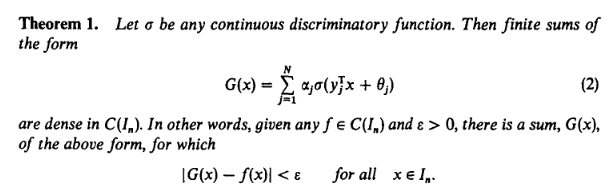The universal approximation theorem in its full glory :) Source: Cybenko, G. (1989) "Approximations by superpositions of sigmoidal functions", Mathematics of Control, Signals, and Systems, 2(4), 303–314.

Don't worry if the exact theorem seems difficult. I will explain the whole process in detail. (In fact, I deliberately skipped concepts like dense to make the explanation clearer, albeit less precise.)

Step one. Suppose that the function to learn is g(x), which is continuous. Let's fix a small number ε and draw an ε wide stripe around the function. The smaller ε is, the better the result will be.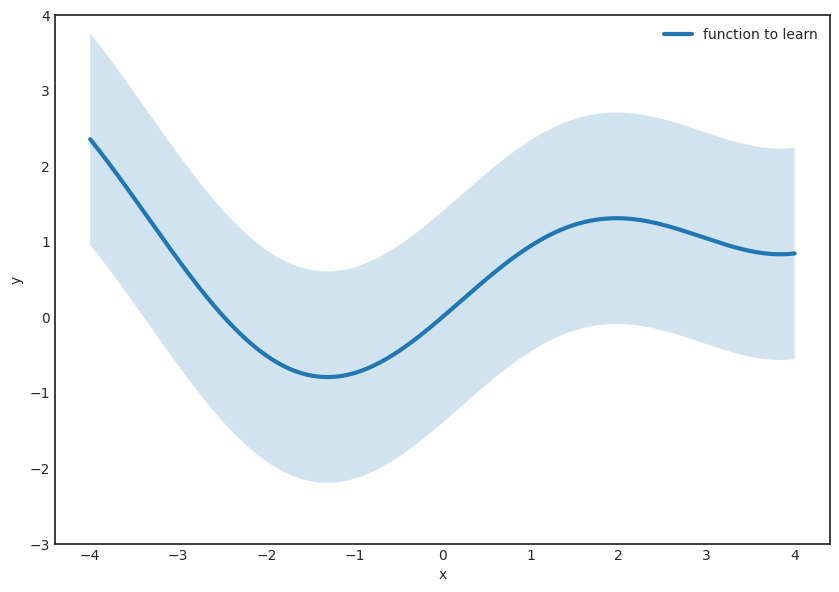Step two. (The hard part.) Find a function of the formthat is completely inside the stripe. The theorem guarantees the existence of such F(x). Hence this family of functions is called universal approximators. This is the awesome thing about neural networks, giving them their real power.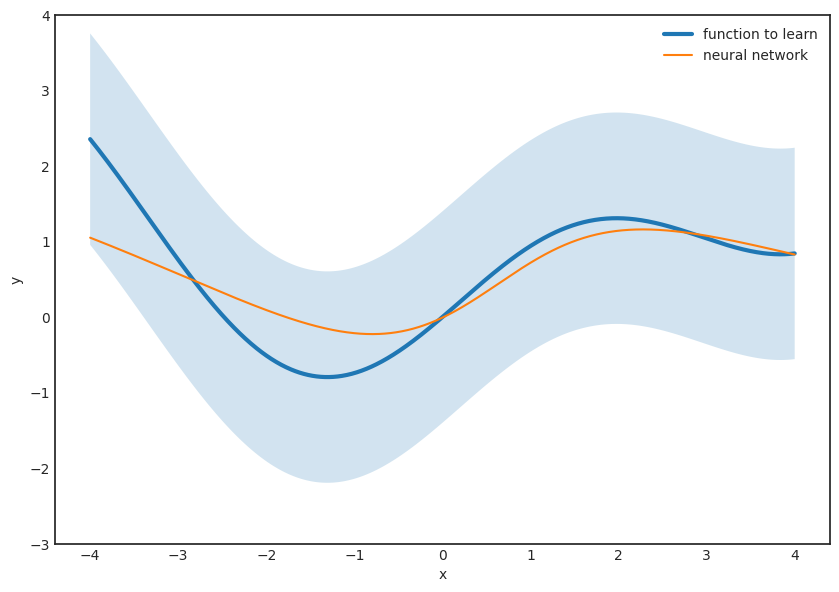There are several caveats, however. For instance, the theorem doesn't say anything about N, the number of neurons in the hidden layer. For small ε, this can be very large, which is bad from a computational perspective. We want to calculate predictions as fast as possible, and calculating the sum of ten billion terms is definitely not fun.

The second issue is that even though the theorem guarantees the existence of a good approximating function, it doesn't tell us how to find it. Although this might be surprising, this is very typical in mathematics. We have extremely powerful tools to reason about the existence of particular objects without constructing them explicitly. (There is a mathematical school called constructivism that rejects purely existence proofs such as the original proof of the universal approximation theorem. However, the problem is very deep-rooted. Without accepting nonconstructive proofs, we wouldn't even be able to talk about functions on infinite sets.)

However, the biggest issue is that in practice, we never know the underlying function completely. We only know what we have observed:There is an infinite number of possible configurations which could fit our data arbitrarily well. Most of them generalize horribly to new data. You undoubtedly know this phenomenon: it is the dreaded overfitting.

## With great power comes great responsibility

So, here is the thing. If you have N observations, you can find a polynomial of degree N-1 that perfectly fits your observations. This is not a big deal. You can even write down this polynomial explicitly by using Lagrange interpolation. However, it won't generalize to any new data. In fact, it will be awful. The figure below demonstrates what happens when we try to fit a large degree polynomial to a small dataset.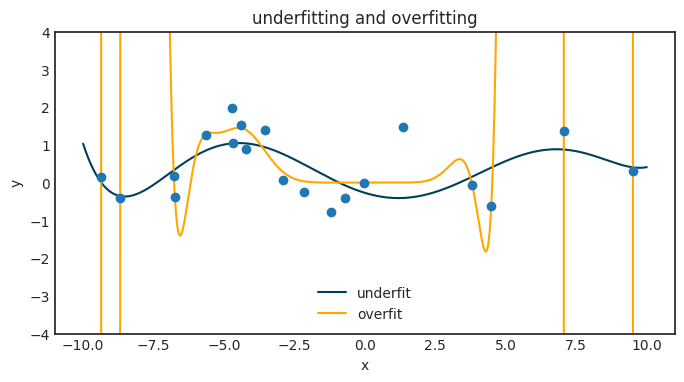The very same phenomenon holds for neural networks. This is a huge problem, and the universal approximation theorem gives us absolutely zero hints on how to overcome this.

In general, the more expressive a function family is, the more it is prone to overfitting. With great power comes great responsibility. This is called the bias-variance tradeoff. For neural networks, there are lots of approaches to mitigate this, from L1 regularization of weights to dropoff layers. However, since neural networks are so expressive, this problem is always looming in the background and requires constant attention.

## Beyond the universal approximation theorem

As I have mentioned, the theorem gives no tools to find a parameter configuration for our neural network. From a practical standpoint, this is almost as important as the universal approximating property. For decades, neural networks were out of favor because of the lack of a computationally effective method to fit them to the data. There were two essential advances, which made their use feasible: backpropagation and general-purpose GPU-s. With these two under your belt, training massive neural networks is a breeze. You can train state-of-the-art models using your notebook without even breaking a sweat. We have come so far since the universal approximation theorem!

Usually, this is the starting point of a standard deep learning course. Due to its mathematical complexity, the theoretical foundations of neural networks are not covered. However, the universal approximation theorem (and the tools used in its proof) gives a profound insight into why neural networks are so powerful. It even lays the groundwork for engineering novel architectures. After all, who said that we are only allowed to combine sigmoids and linear functions?